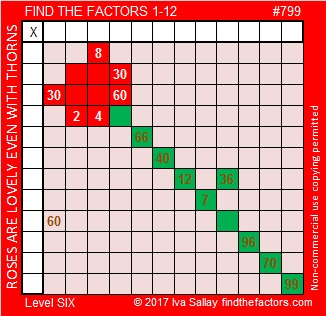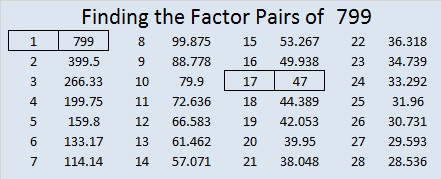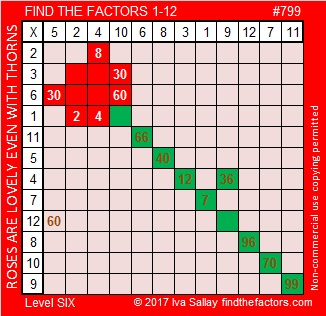# 799 A Rose for Your Valentine

Roses are beautiful and make lovely gifts for Valentines or any other occasion. A Native American legend explains why roses have thorns.

The rose on today’s puzzle has thorns because without thorny clue 60 the puzzle would not have a unique solution. You can be sure that 60 will play an important part in using logic to find the solution to this puzzle.

With or without a valentine, love your brain and give the puzzle a try. It won’t be easy, but you should eventually be able to figure it out. My blogging friend, justkinga, has some other suggestions to show YOURSELF some love on Valentine’s Day.Print the puzzles or type the solution on this excel file: 12-factors-795-799

7 + 9 + 9 = 25, a composite number.

7^3 + 9^3 + 9^3 = 1801, a prime number.

Stetson.edu states that 799 is the smallest number whose digits add up to a composite number AND whose digits cubed add up to a prime number.

It may seem like an improbable number fact, but it wasn’t too difficult to verify, and it really is true!

799 is also the smallest number whose digits add up to 25. (The digits of 889 also add up to 25, and its digits cubed also add up to a prime number. Could this be more than a coincidence?)

Here’s more about the number 799:

799 is palindrome 1H1 in BASE 21 (H is 17 base 10). Note that 1(441) + 17(21) + 1(1) = 799.

799 is the hypotenuse of Pythagorean triple 376-705-799 which is 47 times 8-15-17.

• 799 is a composite number.
• Prime factorization: 799 = 17 x 47
• The exponents in the prime factorization are 1 and 1. Adding one to each and multiplying we get (1 + 1)(1 + 1) = 2 x 2 = 4. Therefore 799 has exactly 4 factors.
• Factors of 799: 1, 17, 47, 799
• Factor pairs: 799 = 1 x 799 or 17 x 47
• 799 has no square factors that allow its square root to be simplified. √799 ≈ 266588.